Download Presentation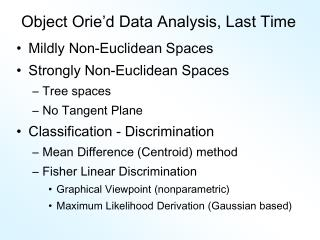Object Orie’d Data Analysis, Last Time

# Object Orie’d Data Analysis, Last Time - PowerPoint PPT Presentation

Object Orie’d Data Analysis, Last Time. Mildly Non-Euclidean Spaces Strongly Non-Euclidean Spaces Tree spaces No Tangent Plane Classification - Discrimination Mean Difference (Centroid) method Fisher Linear Discrimination Graphical Viewpoint (nonparametric)I am the owner, or an agent authorized to act on behalf of the owner, of the copyrighted work described.
Download Presentation## Object Orie’d Data Analysis, Last Time

An Image/Link below is provided (as is) to download presentation

Download Policy: Content on the Website is provided to you AS IS for your information and personal use and may not be sold / licensed / shared on other websites without getting consent from its author.While downloading, if for some reason you are not able to download a presentation, the publisher may have deleted the file from their server.

- - - - - - - - - - - - - - - - - - - - - - - - - - E N D - - - - - - - - - - - - - - - - - - - - - - - - - -
Presentation Transcript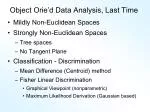Object Orie’d Data Analysis, Last Time
• Mildly Non-Euclidean Spaces
• Strongly Non-Euclidean Spaces
• Tree spaces
• No Tangent Plane
• Classification - Discrimination
• Mean Difference (Centroid) method
• Fisher Linear Discrimination
• Graphical Viewpoint (nonparametric)
• Maximum Likelihood Derivation (Gaussian based)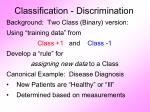Classification - Discrimination

Background: Two Class (Binary) version:

Using “training data” from

Class +1 and Class -1

Develop a “rule” for

assigning new data to a Class

Canonical Example: Disease Diagnosis

• New Patients are “Healthy” or “Ill”
• Determined based on measurements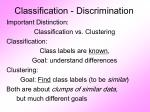Classification - Discrimination

Important Distinction:

Classification vs. Clustering

Classification:

Class labels are known,

Goal: understand differences

Clustering:

Goal: Find class labels (to be similar)

Both are about clumps of similar data,

but much different goals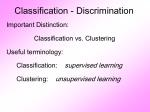Classification - Discrimination

Important Distinction:

Classification vs. Clustering

Useful terminology:

Classification: supervised learning

Clustering: unsupervised learning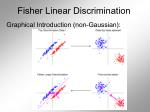Fisher Linear Discrimination

Graphical Introduction (non-Gaussian):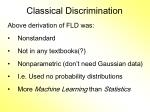Classical Discrimination

Above derivation of FLD was:

• Nonstandard
• Not in any textbooks(?)
• Nonparametric (don’t need Gaussian data)
• I.e. Used no probability distributions
• More Machine Learning than Statistics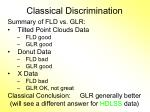Classical Discrimination

Summary of FLD vs. GLR:

• Tilted Point Clouds Data
• FLD good
• GLR good
• Donut Data
• GLR good
• X Data
• GLR OK, not great

Classical Conclusion: GLR generally better

(will see a different answer for HDLSS data)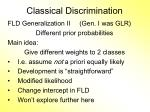Classical Discrimination

FLD Generalization II (Gen. I was GLR)

Different prior probabilities

Main idea:

Give different weights to 2 classes

• I.e. assume not a priori equally likely
• Development is “straightforward”
• Modified likelihood
• Change intercept in FLD
• Won’t explore further here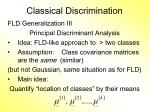Classical Discrimination

FLD Generalization III

Principal Discriminant Analysis

• Idea: FLD-like approach to > two classes
• Assumption: Class covariance matrices are the same (similar)

(but not Gaussian, same situation as for FLD)

• Main idea:

Quantify “location of classes” by their means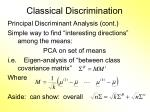Classical Discrimination

Principal Discriminant Analysis (cont.)

Simple way to find “interesting directions” among the means:

PCA on set of means

i.e. Eigen-analysis of “between class covariance matrix”

Where

Aside: can show: overallClassical Discrimination

Principal Discriminant Analysis (cont.)

But PCA only works like Mean Difference,

Expect can improve by

taking covariance into account.

Blind application of above ideas suggests eigen-analysis of:Classical Discrimination

Principal Discriminant Analysis (cont.)

There are:

• smarter ways to compute

(“generalized eigenvalue”)

• other representations

(this solves optimization prob’s)

Special case: 2 classes,

reduces to standard FLD

Good reference for more: Section 3.8 of:

Duda, Hart & Stork (2001)Classical Discrimination

Summary of Classical Ideas:

• Among “Simple Methods”
• MD and FLD sometimes similar
• Sometimes FLD better
• So FLD is preferred
• Among Complicated Methods
• GLR is best
• So always use that
• Caution:
• Story changes for HDLSS settingsHDLSS Discrimination

Recall main HDLSS issues:

• Sample Size, n < Dimension, d
• Singular covariance matrix
• So can’t use matrix inverse
• I.e. can’t standardize (sphere) the data

(requires root inverse covariance)

• Can’t do classical multivariate analysisHDLSS Discrimination

An approach to non-invertible covariances:

• Replace by generalized inverses
• Sometimes called pseudo inverses
• Note: there are several
• Here use Moore Penrose inverse
• As used by Matlab (pinv.m)
• Often provides useful results

(but not always)

Recall Linear Algebra Review…Recall Linear Algebra

Eigenvalue Decomposition:

For a (symmetric) square matrix

Find a diagonal matrix

And an orthonormal matrix

(i.e. )

So that: , i.e.Recall Linear Algebra (Cont.)
• Eigenvalue Decomp. solves matrix problems:
• Inversion:
• Square Root:
• is positive (nonn’ve, i.e. semi) definite allRecall Linear Algebra (Cont.)

Moore-Penrose Generalized Inverse:

ForRecall Linear Algebra (Cont.)
• Easy to see this satisfies the definition of
• Generalized (Pseudo) Inverse
• symmetric
• symmetricRecall Linear Algebra (Cont.)

Moore-Penrose Generalized Inverse:

Idea: matrix inverse on non-null space

of the corresponding linear transformation

Reduces to ordinary inverse, in full rank case,

i.e. for r = d, so could just always use this

Tricky aspect:

“>0 vs. = 0” & floating point arithmeticHDLSS Discrimination

Application of Generalized Inverse to FLD:

Direction (Normal) Vector:

Intercept:

Have replaced byHDLSS Discrimination

Toy Example: Increasing Dimension

data vectors:

• Entry 1:

Class +1: Class –1:

• Other Entries:
• All Entries Independent

Look through dimensions,HDLSS Discrimination

Increasing Dimension Example

Proj. on

Opt’l Dir’n

Proj. on

FLD Dir’n

Proj. on

both Dir’nsHDLSS Discrimination

Add a 2nd Dimension (noise)

Same Proj. on

Opt’l Dir’n

Axes same

as dir’ns

Now See 2

Dim’nsHDLSS Discrimination

Add a 3rd Dimension (noise)

Project on

2-d subspace

generated

by optimal

dir’n & by

FLD dir’nHDLSS Discrimination

Movie Through Increasing DimensionsHDLSS Discrimination

FLD in Increasing Dimensions:

• Low dimensions (d = 2-9):
• Visually good separation
• Small angle between FLD and Optimal
• Good generalizability
• Medium Dimensions (d = 10-26):
• Visual separation too good?!?
• Larger angle between FLD and Optimal
• Worse generalizability
• Feel effect of sampling noiseHDLSS Discrimination

FLD in Increasing Dimensions:

• High Dimensions (d=27-37):
• Much worse angle
• Very poor generalizability
• But very small within class variation
• Poor separation between classes
• Large separation / variation ratioHDLSS Discrimination

FLD in Increasing Dimensions:

• At HDLSS Boundary (d=38):
• 38 = degrees of freedom

(need to estimate 2 class means)

• Within class variation = 0 ?!?
• Data pile up, on just two points
• Perfect separation / variation ratio?
• But only feels microscopic noise aspects

So likely not generalizable

• Angle to optimal very largeHDLSS Discrimination

FLD in Increasing Dimensions:

• Just beyond HDLSS boundary (d=39-70):
• Improves with higher dimension?!?
• Angle gets better
• Improving generalizability?
• More noise helps classification?!?HDLSS Discrimination

FLD in Increasing Dimensions:

• Far beyond HDLSS boun’ry (d=70-1000):
• Projections look terrible

(populations overlap)

• And Generalizability falls apart, as well
• Math’s worked out by Bickel & Levina (2004)
• Problem is estimation of d x d covariance matrixHDLSS Discrimination

Simple Solution:

Mean Difference (Centroid) Method

• Recall not classically recommended
• Usually no better than FLD
• Sometimes worse
• But avoids estimation of covariance
• Means are very stable
• Don’t feel HDLSS problemHDLSS Discrimination

Mean Difference (Centroid) Method

Same

Data,

Movie

over

dim’sHDLSS Discrimination

Mean Difference (Centroid) Method

• Far more stable over dimensions
• Because is likelihood ratio solution

(for known variance - Gaussians)

• Doesn’t feel HDLSS boundary
• Eventually becomes too good?!?

Widening gap between clusters?!?

• Careful: angle to optimal grows
• So lose generalizability

HDLSS data present some odd effects…Maximal Data Piling

Strange FLD effect at HDLSS boundary:

Data Piling:

For each class,

all data

project to

single

valueMaximal Data Piling

What is happening?

• Hard to imagine
• Since our intuition is 3-dim’al
• Came from our ancestors…

Try to understand data piling with

some simple examplesMaximal Data Piling

Simple example (Ahn & Marron 2005):

in

Let be the hyperplane:

• Generated by Class +1
• Which has dimension = 1
• I.e. line containing the 2 points

Similarly, let be the hyperplane

• Generated by Class -1Maximal Data Piling

Simple example: in

Let be

• Parallel shifts of
• So that they pass through the origin
• Still have dimension 1
• But now are subspacesMaximal Data Piling

Simple example: inMaximal Data Piling

Simple example: in

Construction 1:

Let be

• Subspace generated by
• Two dimensional
• Shown as cyan planeMaximal Data Piling

Simple example: in

Construction 1 (cont.):

Let be

• Direction orthogonal to
• One dimensional
• Makes Class +1 Data project to one point
• And Class -1 Data project to one point
• Called Maximal Data Piling DirectionMaximal Data Piling

Simple example: in

Construction 2:

Let be

• Subspaces orthogonal to

(respectively)

• Projection collapses Class +1
• Projection collapses Class -1
• Both are 2-d (planes)Maximal Data Piling

Simple example: in

Construction 2 (cont.):

Let intersection of be

• Same Maximal Data Piling Direction
• Projection collapses both

Class +1 and Class -1

• Intersection of 2-d (planes) is 1-d dir’nMaximal Data Piling

General Case: in with

Let be

• Hyperplanes generated by Classes
• Of Dimensions (resp.)

Let be

• Parallel subspaces
• I.e. shifts to origin
• Of Dimensions (resp.)Maximal Data Piling

General Case: in with

Let be

• Orthogonal Hyperplanes
• Of Dim’ns (resp.)
• Where
• Proj’n in Dir’ns Collapse Class +1
• Proj’n in Dir’ns Collapse Class -1
• Expect intersectionMaximal Data Piling

General Case: in with

Can show (Ahn & Marron 2005):

• Most dir’ns in intersection collapse all to 0
• But there is a direction,
• Where Classes collapse to different points
• Unique in subspace generated by the data
• Called Maximal Data Piling DirectionMaximal Data Piling

Movie Through Increasing DimensionsMaximal Data Piling

FLD in Increasing Dimensions:

• Sub HDLSS dimensions (d = 1-37):
• Looks similar to FLD?!?
• Reason for this?
• At HDLSS Boundary (d = 38):
• Again similar to FLD…Maximal Data Piling

FLD in Increasing Dimensions:

• For HDLSS dimensions (d = 39-1000):
• Always have data piling
• Gap between grows for larger n
• Even though noise increases?
• Angle (gen’bility) first improves, d = 39–180
• Then worsens, d = 200-1000
• Eventually noise dominates
• Trade-off is where gap near optimal diff’nceMaximal Data Piling

How to compute ?

Can show (Ahn & Marron 2005):

Recall FLD formula:

Only difference is global vs. within class

Covariance Estimates!Maximal Data Piling

Historical Note:

• Discovery of MDP
• Came from a programming error
• Forgetting to use within class covariance
• In FLD…Maximal Data Piling

Visual similarity of & ?

Can show (Ahn & Marron 2005), for d<n:

I.e. directions are the same!

• How can this be?
• Note lengths are different…
• Study from transformation viewpointMaximal Data Piling

Recall

Transfo’

view of

FLD:Maximal Data Piling

Include

Corres-

ponding

MDP

Transfo’:

Both give

Same

Result!Maximal Data Piling

Details:

FLD,

sep’ing plane

normal vector

Within Class,

PC1

PC2

Global,

PC1

PC2Maximal Data Piling

Acknowledgement:

• This viewpoint
• I.e. insight into why FLD = MDP

(for low dim’al data)

• Suggested by Daniel PeñaMaximal Data Piling

Fun e.g:

rotate

from PCA

to MDP

dir’nsMaximal Data Piling

MDP for other class labellings:

• Always exists
• Separation bigger for natural clusters
• Could be used for clustering
• Consider all directions
• Find one that makes largest gap
• Very hard optimization problem
• Over 2n possible directionsMaximal Data Piling

A point of terminology (Ahn & Marron 2005):

MDP is “maximal” in 2 senses:

• # of data piled
• Size of gap

(within subspace

gen’d by data)Maximal Data Piling

Recurring, over-arching, issue:

HDLSS space is a weird placeKernel Embedding

Aizerman, Braverman and Rozoner (1964)

• Motivating idea:

Extend scope of linear discrimination,

By adding nonlinear components to data

(embedding in a higher dim’al space)

• Better use of name:

nonlinear discrimination?Kernel Embedding

Toy Examples:

In 1d, linear separation splits the domain

into only 2 partsKernel Embedding

But in the “quadratic

embedded domain”,

linear separation

can give 3 partsKernel Embedding

But in the quadratic embedded domain

Linear separation can give 3 parts

• original data space lies in 1d manifold
• very sparse region of
• curvature of manifold gives:

better linear separation

• can have any 2 break points

(2 points line)Kernel Embedding

Stronger effects for

higher order

polynomial embedding:

E.g. for cubic,

linear separation

can give 4 parts

(or fewer)Kernel Embedding

Stronger effects - high. ord. poly. embedding:

• original space lies in 1-d manifold,

even sparser in

• higher d curvature gives:

improved linear separation

• can have any 3 break points

(3 points plane)?

• Note: relatively few

“interesting separating planes”Kernel Embedding

General View: for original data matrix:

i.e. embed in

Higher

Dimensional

spaceKernel Embedding

EmbeddedFisher Linear Discrimination:

Choose Class 1, for any when:

in embedded space.

• image of class boundaries in original space is nonlinear
• allows more complicated class regions
• Can also do Gaussian Lik. Rat. (or others)
• Compute image by classifying points from original space